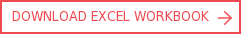View More Content
SHARE

# Circular interest on average balancesCategoriesTutorialsSolve common problems with calculating interest using basic algebra instead of VBA.

You may have encountered a circular reference when writing formulas in Excel. In this tutorial, we demonstrate how to solve a common problem related to interest using high school mathematics rather than visual basic application (VBA). You can download the accompanying Excel workbook which illustrates this tutorial by clicking on the download Excel workbook button.

A circular reference is created when the formula is directly or indirectly dependent on itself. Circular logic is when C=A+B but in turn A or B is a function of C.

Although it is possible to solve, using an iterative approach, this breaks a fundamental rule in efficient financial modelling. The widely used solution in the market is strewn with shortcomings. That’s why we decided to demonstrate how we have solved this problem using a simple algebra formula.Solution: Solving circular interest with algebra

## Why are circular references a bad idea?

In this tutorial, we will look at interest on a deposit or a loan account, where the interest is calculated on the average balance and the interest accumulates within the account, e.g., during construction period. This is a simple situation, but it has stumped the finance industry for the past several decades.

The interest can readily be calculated in a spreadsheet by accommodating a circular reference however this action has several consequences:

• It breaks a firm rule of best practice modelling - 'no circular references'
• Reputable model review firms will qualify their formal opinion
• It breaches the in-house modelling risk policies in most reputable institutions

## Alternative approach to solving circular interest

The problem can be solved, albeit crudely, by isolating the circular reference using a copy-and-paste macro. This involves copying the calculated interest and pasting it into the account, where upon the interest calculation is updated and the loop happens once more.

This is performed until the difference between the 'calculated interest' and the 'value copied interest' is below a level of tolerance in all periods. As soon as the model parameters change, this macro will need to be run again. This is the general practice in the market; however, it has the following additional drawbacks:

• It cannot be used readily with 'data tables' – a powerful scenario tool
• It relies on the user to know when and how to execute the macro
• It relies on ‘solid’ VBA coding otherwise the cell references may move

Like many calculations found in finance there is a simple way and a hard way. Reflecting on what you are really trying to solve usually leads to a more elegant analytical rather than iterative approach.

## Using algebra to solve circular interest

A circular reference is formed when interest is a function of the average balance of the loan. Consider this:

• CB = Closing balance
• OB = Opening balance
• I = Interest earned
• r = Deposit interest rateand we have:The above formulae can be rearranged by simultaneous substitution, so the algebraic solution for interest is:To demonstrate this method we have included a simple workbook example where the interest on the debt account is calculated on the average balance and the interest accumulates within the debt account.

To illustrate the problem above, we solve the interest in this example both with a traditional iterative routine and then algebraically. You will see in the attached workbook that they yield the same result. Withdrawals from and deposits to the account can also be incorporated using a similar approach. Download the Excel workbook to see more.

## Corality Training Academy - SMART Campus